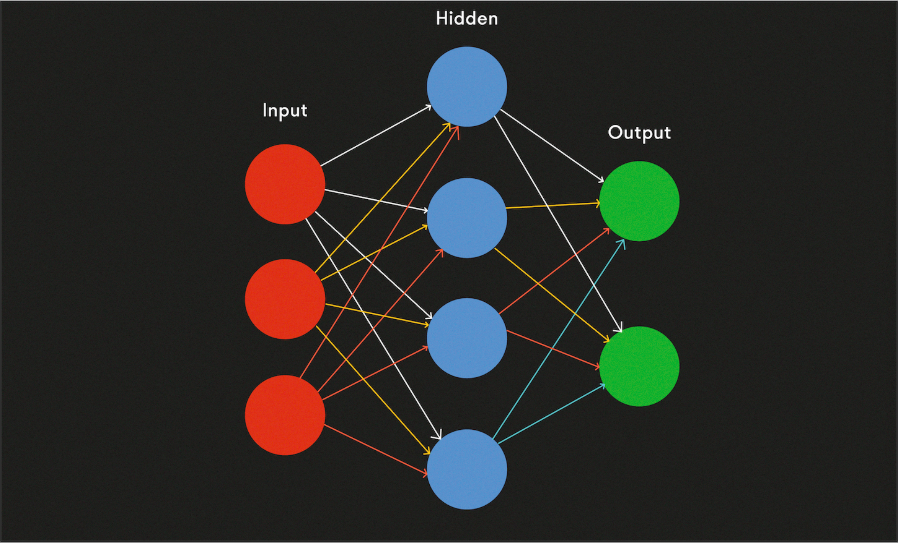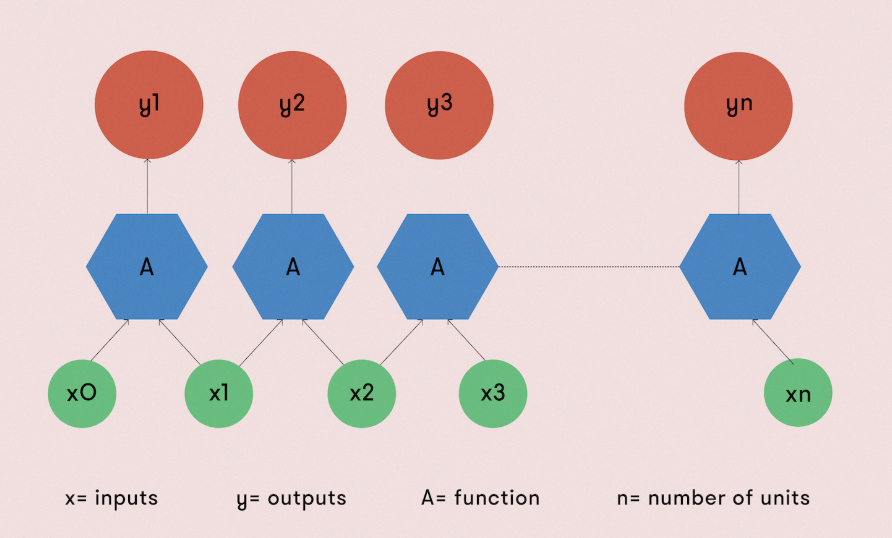## 为什么函数式编程更适合深度学习## Clojure

### Clojure 中强大的并发编程

Clojure 并非 Java 线程系统的替代品，而是与后者协同工作。由于核心数据结构是不可变的，因此可以在线程之间轻松地共享它们。与此同时，程序中的状态可以更改的，Clojure 提供了确保状态一致性的机制。如果两个事务在试图修改相同引用时发生冲突，其中一个将退出。这里我们不需要显式锁定。

import ‘(java.util.concurrent Executors))(defn test-stm [nitems nthreads niters](let [refs (map ref (repeat nitems 0))   pool (Executors/newFixedThreadPool nthreads)   tasks (map (fn [t]               (fn []                (dotimes [n niters]                  (dosync                   (doseq [r refs]                    (alter r + 1 t))))))              (range nthreads))]   (doseq [future (.invokeAll pool tasks)]      (.get future))   (.shutdown pool)   (map deref refs)))(test-stm 10 10 10000) -> (550000 550000 550000 550000 550000 550000 550000 550000 550000 550000)

### Clojure 中的并行性很廉价

(defn calculate-pixels-2 [] (let [n (* *width* *height*)       work (partition (/ n 16) (range 0 n))       result (pmap (fn [x]                  (doall (map                   (fn [p]                     (let [row (rem p *width*) col (int (/ p *height*))]                       (get-color (process-pixel (/ row (double *width*)) (/ col (double *height*))))))                   x)))                  work)]   (doall (apply concat result))))

### Clojure 中的链式函数意味着更清晰的代码

;; pipe arg to function(-> "x" f1) ; "x1" ;; pipe. function chaining(-> "x" f1 f2) ; "x12"

### 库和局限性

import Numeric.LinearAlgebra.Static.Backproplogistic :: Floating a => a -> alogistic x = 1 / (1 + exp (-x))feedForwardLog   :: (KnownNat i, KnownNat o)   => Model (L o i :& R o) (R i) (R o)feedForwardLog (w :&& b) x = logistic (w #> x + b)